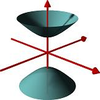#### You may also like### A Close Match

Can you massage the parameters of these curves to make them match as closely as possible?### Prime Counter

A short challenge concerning prime numbers.### The Right Volume

Can you rotate a curve to make a volume of 1?

# Power Stack

##### Age 16 to 18 ShortChallenge Level
Kimberly wants to define $3^{3^3}$ as $(3^3)^3$ but Nermeen thinks that such a stack of powers should be defined as $3^{(3^3)}$ .

Do their definitions lead to the same numerical value? Is the same true if $3$ is replaced with some other number?

How would Kimberly's and Nermeen's definitions most naturally extend to the definition of $3^{3^{3^3}}$? Do their definitions lead to the same numerical value? Is the same true if $3$ is replaced with some other number?

Extension: Try to compute the approximate size of the numbers as powers of 10.

Did you know ... ?

Both definitions of powers are equally valid, and in mathematics it should be clear from the context as to which to apply: mathematicians often include the brackets to avoid ambiguity. Kimberly's definition of powers is often relevant in mathematics problems whereas Nermeen's definition of powers is often relevant in computer science problems.